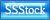## Add My Banner!!## - Friends Link -## Saturday, February 21, 2009

### COMMODITY CHANNEL INDEX

Commodity Channel Index (CCI) created by Donald Lambert. It measures the variation of a security's price from its statistical mean. High values show that prices are unusually high compared to average prices whereas low values indicate that prices are unusually low.

The CCI typically oscillates between ±100. To use the CCI as an overbought/oversold indicator, readings above +100 imply an overbought condition (and a pending price correction) while readings below -100 imply an oversold condition (and a pending rally).

A complete explanation of the CCI calculation is beyond the scope of this book.

The following are basic steps involved in the calculation:

1.Add each period's high, low, and close and divide this sum by 3.

2.Calculate an n-period simple moving average of the typical prices computed in Step 1.

3.For each of the prior n-periods, subtract today's Step 2 value from Step 1's value n days ago. For example, if you were calculating a 5-day CCI, you would perform five subtractions using today's Step 2 value.

4.Calculate an n-period simple moving average of the absolute values of each of the results in Step 3.

5. Multiply the value in Step 4 by 0.015.

6. Subtract the value from Step 2 from the value in Step 1.

7. Divide the value in Step 6 by the value in Step 5.